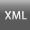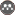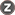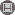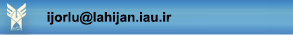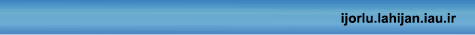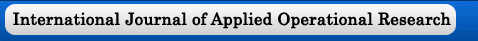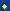homesubmit paperguide for authorscontact usregistersearcharchivecurrent issuejournal info[Home ] [Archive]Volume 9, Issue 1 (Special Issue on the 9th International Conference of Fuzzy Information and Engineering 2019)2019, 9(1): 47-52 Back to browse issues page
On the hybrid conjugate gradient method for solving fuzzy optimization problem
M. Ranjbar , Z. Akbari
Department of Mathematics, University of Mazandaran, Babolsar, Iran
Abstract:   (894 Views)
In this paper we consider a constrained optimization problem where the objectives are fuzzy functions (fuzzy-valued functions). Fuzzy constrained Optimization (FO) problem plays an important role in many fields, including mathematics, engineering, statistics and so on. In the other side, in the real situations, it is important to know how may obtain its numerical solution of a given interesting problem. In this paper we defined Necessary and sufficient optimality conditions for constrained fuzzy optimization problem and a Hybrid Conjugate Gradient (HCG) algorithm was proposed for solving FOP. The parametric form of the function was translated into an equivalent Fuzzy constrained Optimization (FO) problem. Then, HCG algorithm was applied to solve the corresponding optimization problem.

Keywords: Fuzzy constrained Optimization, Hybrid Conjugate Gradient Algorithm, constrained Optimization Problem, Steepest Descent Algorithm, Generalized Hukuhara differentiability
Type of Study: Research | Subject: Special